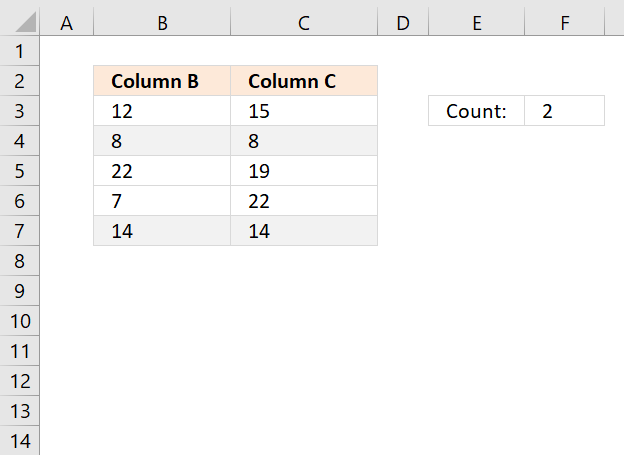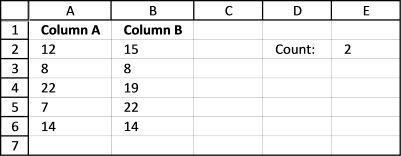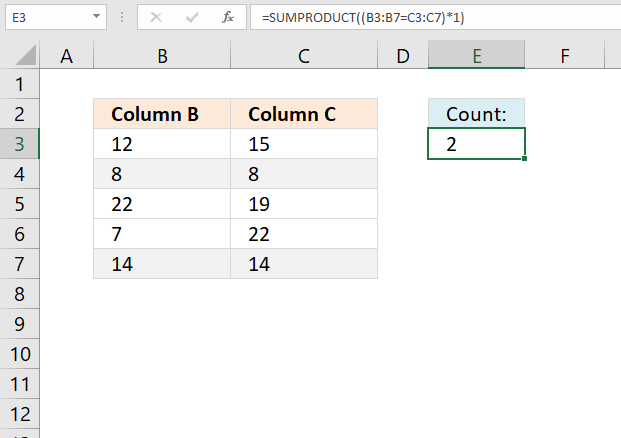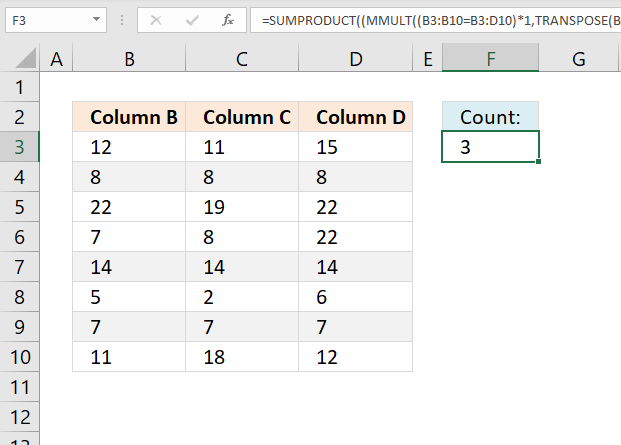Author: Oscar Cronquist Article last updated on June 02, 2021This article describes a formula that counts values in two columns if they are duplicates on the same row.

## 1. Count identical values if they are on the same row (Array formula)

Hi Oscar,Need a formula to count identical numbers in two columns but items must be in same row (position).

12 15
8 8 good count 1
22 19
7 22 for 22 not count cause is not in same row
14 14 good count 2Array formula in cell E2:

=SUM((A2:A6=B2:B6)*1)

### 1.1 How to enter an array formula

1. Select cell E2
2. Paste the formula in formula bar
3. Press and hold CTRL + SHIFT simultaneously
4. Press Enter
5. Release all keys

Your formula now begins and ends with a curly bracket, if you did it right.

Like this {=SUM((A2:A6=B2:B6)*1)}

Don't enter the curly brackets yourself, they appear automatically.

### 1.2 Explaining formula

#### Step 1 - Compare values in column A with column B

The equal sign lets you compare value to value, the result is boolean value TRUE if they match (not case-sensitive) and FALSE if they don't.

A2:A6=B2:B6

becomes

{12; 8; 22; 7; 14}={15; 8; 19; 22; 14}

and returns

{FALSE; TRUE; FALSE; FALSE; TRUE}

#### Step 2 - Multiply boolean values with 1

To be able to sum the values in this array {FALSE; TRUE;FALSE;FALSE;TRUE} we need to convert the boolean values to their numerical equivalents, FALSE = 0 (zero) and TRUE = 1.

(A2:A6=B2:B6)*1

becomes

({FALSE;TRUE;FALSE;FALSE;TRUE})*1

and returns

{0; 1; 0; 0; 1}

#### Step 3 - Sum values in array

The SUM function adds all numbers in the array and returns a total.

SUM((A2:A6=B2:B6)*1)

becomes

SUM({0; 1; 0; 0; 1})

and returns 2.

### 1.3 Trim space characters

This formula also works with text values, to remove blanks before and after use TRIM function.

=SUM((TRIM(A2:A6)=TRIM(B2:B6))*1)

## 2. Count identical values if they are on the same row (Regular formula)=SUMPRODUCT((B3:B7=C3:C7)*1)

## 3. Count identical values on the same row comparing values in n columnsThe formula in cell F3 counts the number of rows that contain the same value. In the example shown in the image above row 4, 7, and 9 contain the same value and the formula returns 3.

Note that this formula works with any cell range size, it does not need to be exactly three columns for this formula to work. However, you need to adjust the cell references accordingly in order to get a correct result.

=SUMPRODUCT((MMULT((B3:B10=B3:D10)*1,TRANSPOSE(COLUMN(B3:D10)^0))=COLUMNS(B3:D10))*1)

### 3.1 Explaining formula

#### Step 1 - Compare values across columns

The equal sign lets you check if cell values match, the equal sign is a logical operator and returns a boolean (logical) value. TRUE if they match and FALSE if not.

B3:B10=B3:D10

becomes

{12; 8; 22; 7; 14; 5; 7; 11}={12,11,15; 8,8,8; 22,19,22; 7,8,22; 14,14,14; 5,2,6; 7,7,7; 11,18,12}

and returns

{TRUE, FALSE, FALSE;TRUE, TRUE, TRUE;TRUE, FALSE, TRUE;TRUE, FALSE, FALSE;TRUE, TRUE, TRUE;TRUE, FALSE, FALSE;TRUE, TRUE, TRUE;TRUE, FALSE, FALSE}

#### Step 2 - Convert boolean values

The MMULT function can't handle boolean values, we need to convert TRUE and FALSE to their numerical equivalents. TRUE - 1 and FALSE 0 (zero).

(B3:B10=B3:D10)*1

becomes

{TRUE, FALSE, FALSE;TRUE, TRUE, TRUE;TRUE, FALSE, TRUE;TRUE, FALSE, FALSE;TRUE, TRUE, TRUE;TRUE, FALSE, FALSE;TRUE, TRUE, TRUE;TRUE, FALSE, FALSE}*1

and returns

{1, 0, 0;1, 1, 1;1, 0, 1;1, 0, 0;1, 1, 1;1, 0, 0;1, 1, 1;1, 0, 0}

#### Step 3 - Calculate column numbers

The COLUMN function calculates the column numbers based on a cell reference.

COLUMN(B3:D10)

returns {2, 3, 4}.

Column B is 2, C is 3 and D is column number 4.

#### Step 4 - Convert all numbers to number 1

This step converts all column numbers to number 1. This is done by taking each number in the array raised to the 0 (zero) power.

COLUMN(B3:D10)^0

becomes

{2, 3, 4}^0

and returns {1, 1, 1}.

#### Step 5 - Convert a horizontal range to a vertical range

The TRANSPOSE function allows you to convert a vertical range to a horizontal range, or vice versa.

TRANSPOSE(COLUMN(B3:D10)^0)

becomes

TRANSPOSE({1, 1, 1})

and returns {1; 1; 1}.

The colon and semicolon tell you if an array is arranged vertically or horizontally. This is determined by your computer's regional settings.

#### Step 6 - Calculate the number of matches per row

The MMULT function calculates the matrix product of two arrays, an array as the same number of rows as array1 and columns as array2.

MMULT(array1array2)

MMULT((B3:B10=B3:D10)*1,TRANSPOSE(COLUMN(B3:D10)^0))

becomes

MMULT({1, 0, 0;1, 1, 1;1, 0, 1;1, 0, 0;1, 1, 1;1, 0, 0;1, 1, 1;1, 0, 0}, {1; 1; 1})

and returns {1; 3; 2; 1; 3; 1; 3; 1}.

#### Step 7 - Check if the number of matches is equal to the number of columns

MMULT((B3:B10=B3:D10)*1,TRANSPOSE(COLUMN(B3:D10)^0))=COLUMNS(B3:D10)

becomes

{1; 3; 2; 1; 3; 1; 3; 1}=3

and returns

{FALSE; TRUE; FALSE; FALSE; TRUE; FALSE; TRUE; FALSE}.

#### Step 8 - Convert boolean values

The SUMPRODUCT function can't work with boolean values, we need to convert them to their numerical equivalents. TRUE - 1 and FALSE - 0 (zero).

(MMULT((B3:B10=B3:D10)*1,TRANSPOSE(COLUMN(B3:D10)^0))=COLUMNS(B3:D10))*1

becomes

{FALSE; TRUE; FALSE; FALSE; TRUE; FALSE; TRUE; FALSE}*1

and returns {0; 1; 0; 0; 1; 0; 1; 0}.

SUMPRODUCT((MMULT((B3:B10=B3:D10)*1,TRANSPOSE(COLUMN(B3:D10)^0))=COLUMNS(B3:D10))*1)

becomes

SUMPRODUCT({0; 1; 0; 0; 1; 0; 1; 0})

and returns 3.